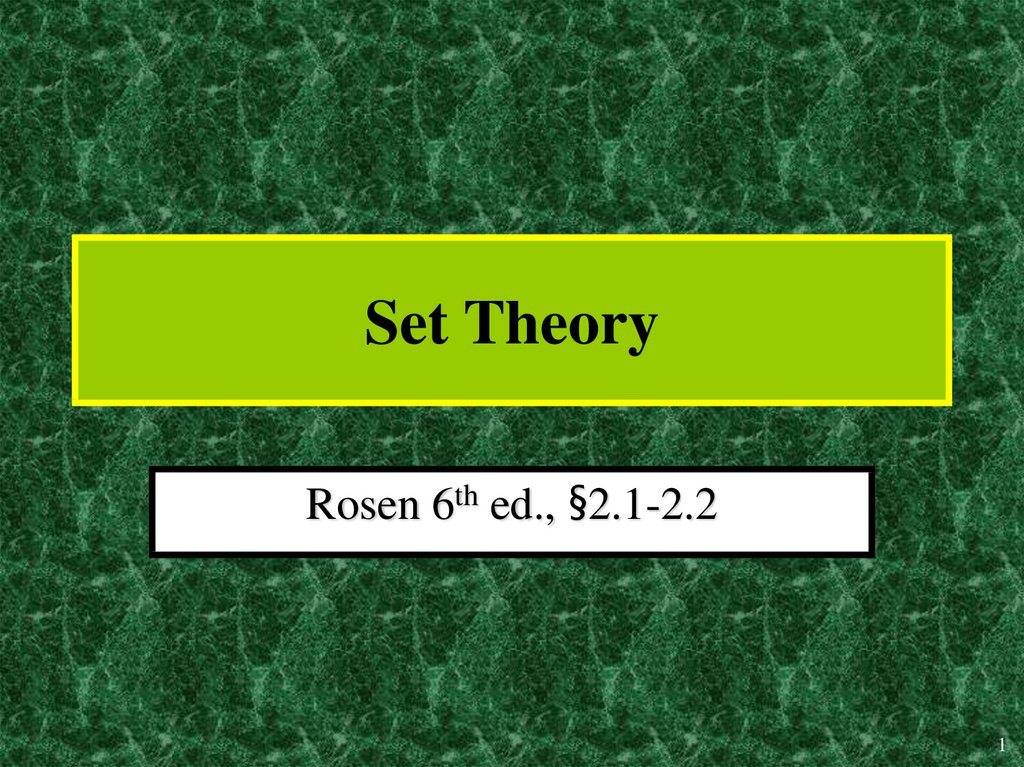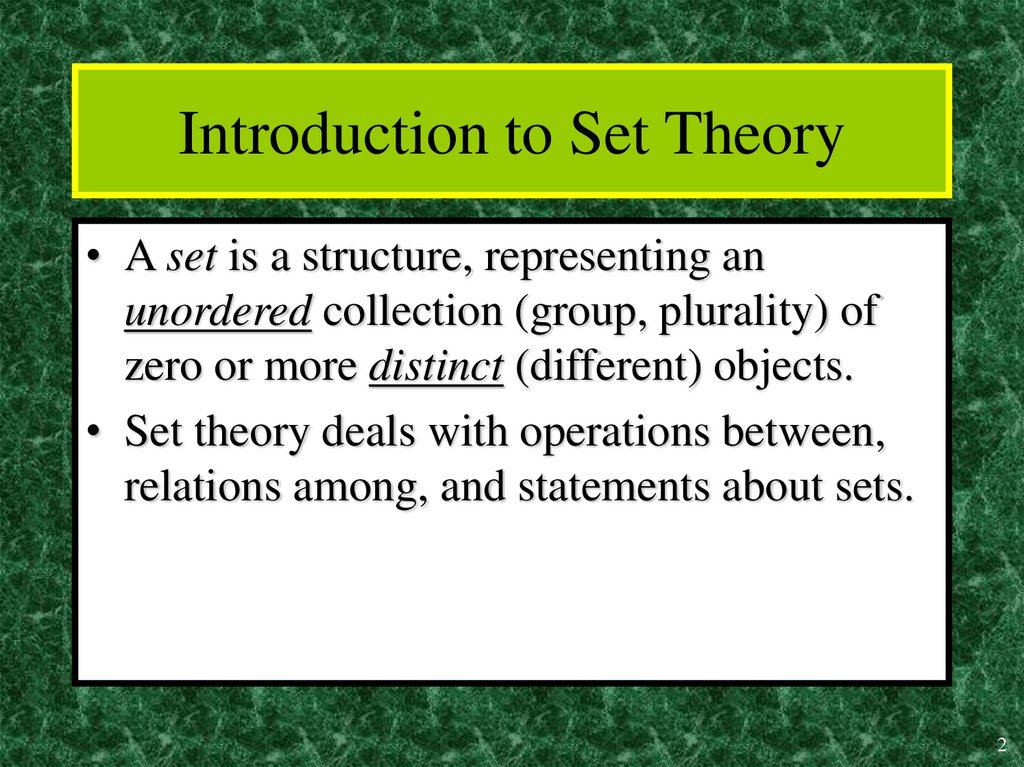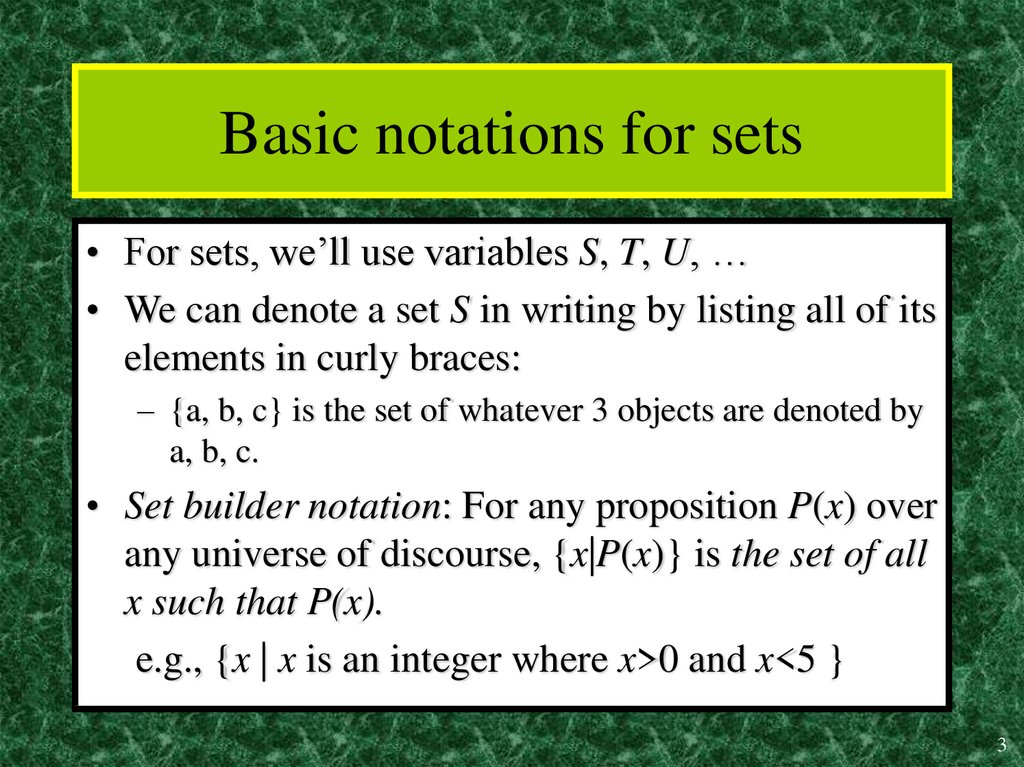# Set Theory

## 1. Set Theory

Rosen 6th ed., §2.1-2.2
1

## 2. Introduction to Set Theory

• A set is a structure, representing an
unordered collection (group, plurality) of
zero or more distinct (different) objects.
• Set theory deals with operations between,
relations among, and statements about sets.
2

## 3. Basic notations for sets

• For sets, we’ll use variables S, T, U, …
• We can denote a set S in writing by listing all of its
elements in curly braces:
– {a, b, c} is the set of whatever 3 objects are denoted by
a, b, c.
• Set builder notation: For any proposition P(x) over
any universe of discourse, {x|P(x)} is the set of all
x such that P(x).
e.g., {x | x is an integer where x>0 and x<5 }
3

## 4. Basic properties of sets

• Sets are inherently unordered:
– No matter what objects a, b, and c denote,
{a, b, c} = {a, c, b} = {b, a, c} =
{b, c, a} = {c, a, b} = {c, b, a}.
• All elements are distinct (unequal);
multiple listings make no difference!
– {a, b, c} = {a, a, b, a, b, c, c, c, c}.
– This set contains at most 3 elements!
4

## 5. Definition of Set Equality

• Two sets are declared to be equal if and only if
they contain exactly the same elements.
• In particular, it does not matter how the set is
defined or denoted.
• For example: The set {1, 2, 3, 4} =
{x | x is an integer where x>0 and x<5 } =
{x | x is a positive integer whose square
is >0 and <25}
5

## 6. Infinite Sets

• Conceptually, sets may be infinite (i.e., not
finite, without end, unending).
• Symbols for some special infinite sets:
N = {0, 1, 2, …} The natural numbers.
Z = {…, -2, -1, 0, 1, 2, …} The integers.
R = The “real” numbers, such as
374.1828471929498181917281943125…
• Infinite sets come in different sizes!
6

7

## 8. Basic Set Relations: Member of

• x S (“x is in S”) is the proposition that object x is
an lement or member of set S.
– e.g. 3 N, “a” {x | x is a letter of the alphabet}
• Can define set equality in terms of relation:
S,T: S=T ( x: x S x T)
“Two sets are equal iff they have all the same
members.”
• x S : (x S)
“x is not in S”
8

## 9. The Empty Set

• (“null”, “the empty set”) is the unique set
that contains no elements whatsoever.
• = {} = {x|False}
• No matter the domain of discourse,
we have the axiom
x: x .
9

## 10. Subset and Superset Relations

• S T (“S is a subset of T”) means that every
element of S is also an element of T.
• S T x (x S x T)
• S, S S.
• S T (“S is a superset of T”) means T S.
• Note S=T S T S T.
• S / T means (S T), i.e. x(x S x T)
10

## 11. Proper (Strict) Subsets & Supersets

Proper (Strict) Subsets & Supersets
• S T (“S is a proper subset of T”) means that
S T but
for S T.
T. /Similar
S
Example:
{1,2}
{1,2,3}
S
T
Venn Diagram equivalent of S T
11

## 12. Sets Are Objects, Too!

• The objects that are elements of a set may
themselves be sets.
• E.g. let S={x | x {1,2,3}}
then S={ ,
{1}, {2}, {3},
{1,2}, {1,3}, {2,3},
{1,2,3}}
• Note that 1 {1} {{1}} !!!!
12

## 13. Cardinality and Finiteness

• |S| (read “the cardinality of S”) is a measure
of how many different elements S has.
• E.g., | |=0, |{1,2,3}| = 3, |{a,b}| = 2,
|{{1,2,3},{4,5}}| = ____
• We say S is infinite if it is not finite.
• What are some infinite sets we’ve seen?
13

## 14. The Power Set Operation

• The power set P(S) of a set S is the set of all
subsets of S. P(S) = {x | x S}.
• E.g. P({a,b}) = { , {a}, {b}, {a,b}}.
• Sometimes P(S) is written 2S.
Note that for finite S, |P(S)| = 2|S|.
• It turns out that |P(N)| > |N|.
There are different sizes of infinite sets!
14

## 15. Cartesian Products of Sets

• For sets A, B, their Cartesian product
A B : {(a, b) | a A b B }.
• E.g. {a,b} {1,2} = {(a,1),(a,2),(b,1),(b,2)}
• Note that for finite A, B, |A B|=|A||B|.
• Note that the Cartesian product is not
commutative: AB: A B =B A.
• Extends to A1 A2 … An...
15

## 16. The Union Operator

• For sets A, B, their union A B is the set
containing all elements that are either in A,
or (“ ”) in B (or, of course, in both).
• Formally, A,B: A B = {x | x A x B}.
• Note that A B contains all the elements of
A and it contains all the elements of B:
A, B: (A B A) (A B B)
16

## 17. Union Examples

• {a,b,c} {2,3} = {a,b,c,2,3}
• {2,3,5} {3,5,7} = {2,3,5,3,5,7} ={2,3,5,7}
17

## 18. The Intersection Operator

• For sets A, B, their intersection A B is the
set containing all elements that are
simultaneously in A and (“ ”) in B.
• Formally, A,B: A B {x | x A x B}.
• Note that A B is a subset of A and it is a
subset of B:
A, B: (A B A) (A B B)
18

## 19. Intersection Examples

• {a,b,c} {2,3} = ___
• {2,4,6} {3,4,5} = ______
{4}
19

## 20. Disjointedness

• Two sets A, B are called
disjoint (i.e., unjoined)
iff their intersection is
empty. (A B= )
• Example: the set of even
integers is disjoint with
the set of odd integers.
Help, I’ve
been
disjointed!
20

## 21. Inclusion-Exclusion Principle

• How many elements are in A B?
|A B| = |A| |B| |A B|
• Example:
{2,3,5} {3,5,7} = {2,3,5,3,5,7} ={2,3,5,7}
21

## 22. Set Difference

• For sets A, B, the difference of A and B,
written A B, is the set of all elements that
are in A but not B.
• A B : x x A x B
x x A x B
• Also called:
The complement of B with respect to A.
22

## 23. Set Difference Examples

• {1,2,3,4,5,6} {2,3,5,7,9,11} =
___________
{1,4,6}
• Z N {… , -1, 0, 1, 2, … } {0, 1, … }
= {x | x is an integer but not a nat. #}
= {x | x is a negative integer}
= {… , -3, -2, -1}
23

## 24. Set Difference - Venn Diagram

• A-B is what’s left after B
“takes a bite out of A”
Chomp!
Set
A B
Set A
Set B
24

## 25. Set Complements

• The universe of discourse can itself be
considered a set, call it U.
• The complement of A, written A, is the
complement of A w.r.t. U, i.e., it is U A.
• E.g., If U=N,
{3,5} {0,1,2,4,6,7,...}
25

## 26. More on Set Complements

• An equivalent definition, when U is clear:
A {x | x A}
A
A
U
26

## 27. Set Identities

Identity:
A =A A U=A
Domination: A U=U A =
Idempotent: A A = A = A A
Double complement: ( A ) A
Commutative: A B=B A A B=B A
Associative: A (B C)=(A B) C
A (B C)=(A B) C
27

## 28. DeMorgan’s Law for Sets

• Exactly analogous to (and derivable from)
DeMorgan’s Law for propositions.
A B A B
A B A B
28

## 29. Proving Set Identities

To prove statements about sets, of the form
E1 = E2 (where Es are set expressions), here
are three useful techniques:
• Prove E1 E2 and E2 E1 separately.
• Use logical equivalences.
• Use a membership table.
29

## 30. Method 1: Mutual subsets

Example: Show A (B C)=(A B) (A C).
• Show A (B C) (A B) (A C).
– Assume x A (B C), & show x (A B) (A C).
– We know that x A, and either x B or x C.
• Case 1: x B. Then x A B, so x (A B) (A C).
• Case 2: x C. Then x A C , so x (A B) (A C).
– Therefore, x (A B) (A C).
– Therefore, A (B C) (A B) (A C).
• Show (A B) (A C) A (B C). …
30

## 31. Method 3: Membership Tables

• Just like truth tables for propositional logic.
• Columns for different set expressions.
• Rows for all combinations of memberships
in constituent sets.
• Use “1” to indicate membership in the
derived set, “0” for non-membership.
• Prove equivalence with identical columns.
31

## 32. Membership Table Example

Prove (A B) B = A B.
A
0
0
1
1
B A B (A B) B A B
0
0
0
0
1
1
0
0
0
1
1
1
1
1
0
0
32

## 33. Membership Table Exercise

Prove (A B) C = (A C) (B C).
AB
0 0
0 0
0 1
0 1
1 0
1 0
1 1
1 1
C A B (A B) C A C
0
1
0
1
0
1
0
1
B C
(A C) (B C)
33

## 34. Generalized Union

• Binary union operator: A B
• n-ary union:
A A2 … An : ((…((A1 A2) …) An)
(grouping & order is irrelevant)
n
• “Big U” notation:
A
i
i 1
• Or for infinite sets of sets:
A
A X
34

## 35. Generalized Intersection

• Binary intersection operator: A B
• n-ary intersection:
A A2 … An ((…((A1 A2) …) An)
(grouping & order is irrelevant)
n
• “Big Arch” notation:
A
i 1
• Or for infinite sets of sets:
i
A
A X
35